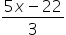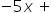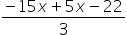Mathematics
Easy

Question

# Solve the following using the substitution method and find the value of x and y:-5x + 3y = -22-5x + y = -24

## 5, 15, 25, 35, 9Hint:

## The correct answer is: 5, 1

### Step 1 of 1:We have given two equations-5x + 3y = -22....(i)-5x + y = -24......(ii)Rewrite first equation and then substitute3y = 5x – 22y=Now substitute the value of y in 2nd equation.= -24= -24-10x – 22 = -72-10x = -50x = 5Now put the value of x in 2nd equation-5(5) + y = -24y = -24 + 25=1

In the substitution method, we first write one variable in the form of another variable.

### Related Questions to study#### With Turito Foundation.#### Get an Expert Advice From Turito.